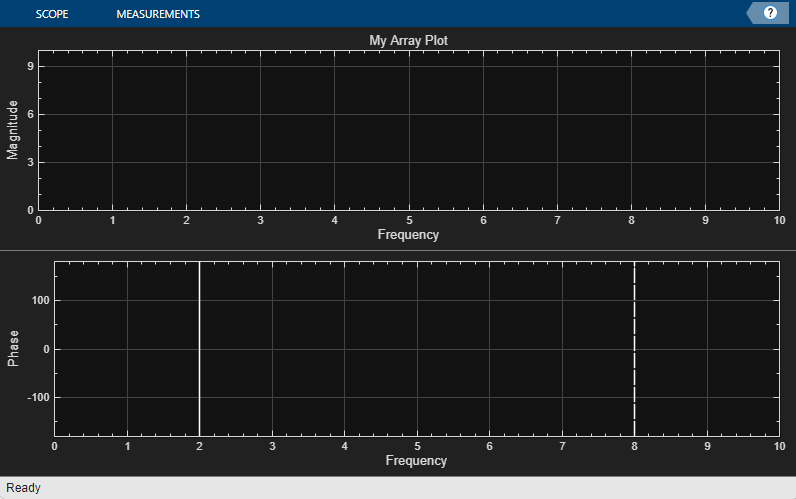# generateScript

Generate MATLAB script to create scope with current settings

## Syntax

``generateScript(scope)``

## Description

````generateScript(scope)` generates a MATLAB® script that can recreate a scope object with the current settings in the scope. ```

## Examples

collapse all

1. Create a `spectrumAnalyzer` object.

```scope = spectrumAnalyzer(); show(scope);```
2. Set options in the Spectrum Analyzer. For this example, enable both Spectrum and Spectrogram to be shown in the Analyzer tab of the Spectrum Analyzer toolstrip.In addition, enable Max-Hold Trace and Min-Hold Trace in the Spectrum tab.3. Generate a script to recreate the `spectrumAnalyzer` with the modified settings. Either click from the Analyzer tab, or enter this command in the MATLAB command prompt.

`generateScript(scope);`

A new editor window opens with code to regenerate the same scope.

```% Generated by MATLAB(R) 9.12 (R2022a) and DSP System Toolbox 9.14 (R2022a). % Generated on 15-Nov-2021 15:24:16 -0500. % Construct a spectrumAnalyzer object specScope = spectrumAnalyzer(ViewType="spectrum-and-spectrogram", ... PlotMaxHoldTrace=true, ... PlotMinHoldTrace=true, ... Position=[184 190 742 563]);```

1. Create a `dsp.ArrayPlot` object.

```scope = dsp.ArrayPlot(); show(scope);```
2. Set options in the Array Plot. For this example, from the Measurements tab, turn on the . From the Plot tab, turn on and . Click and give the plot an XLabel and Title.3. Generate a script to recreate the `dsp.ArrayPlot` with the same modified settings. Either select from the Plot tab, or enter the following in the MATLAB command prompt:

`generateScript(scope);`

A new editor window opens with code to regenerate the same scope.

```% Generated by MATLAB(R) 9.12 (R2022a) and DSP System Toolbox 9.14 (R2022a). % Generated on 8-Dec-2021 15:37:21 -0500. % Construct a dsp.ArrayPlot object arrayPlot = dsp.ArrayPlot(PlotAsMagnitudePhase=true, ... Title="My Array Plot", ... XLabel="Frequency", ... ShowLegend=true); % Cursor Measurements Configuration arrayPlot.CursorMeasurements.Enabled = true;```

1. Create a `timescope` object.

```scope = timescope; show(scope)```
2. Set options in the Time Scope. For this example, on the Scope tab, click Settings. Under Display and Labels, select Show Legend and Magnitude Phase Plot. Set the Title as well.3. Generate a script to recreate the `timescope` with the same modified settings. Either select from the Scope tab, or enter the following in the MATLAB command prompt:

`generateScript(scope);`

A new editor window opens with code to regenerate the same scope.

```% Generated by MATLAB(R) 9.12 (R2022a) and DSP System Toolbox 9.14 (R2022a). % Generated on 8-Dec-2021 15:39:35 -0500. % Construct a timescope object timeScope = timescope(PlotAsMagnitudePhase=true, ... Title="My Time Scope", ... ShowLegend=true);```

## Input Arguments

collapse all

Scope object whose settings you want to recreate with a script, specified as one of the following:

• `spectrumAnalyzer` object

• `dsp.ArrayPlot` object

• `timescope` object

## Version History

Introduced in R2019a

expand all

Not recommended starting in R2022a